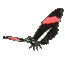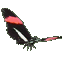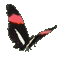Slope from two Ordered Pairs on a Graph      Game Tips:

- The slope from a point P(-2,5) to a point Q(4,-1) is the (rise)/(run) = ((-1)-(5))/(4-(-2)) = (-6)/(6) = -1.
If the run = 0, then the vertical slope is said to be undefined.

- Positive slopes rise to the right. Negative slopes rise to the left.

- A slope of zero is a horizontal slope.

- The slope from a point P(x1,y1) to a point Q(x2,y2) = (change in y)/(change in x) = (y2-y1)/(x2-x1) .

- Your Game Score is reduced by the number of butterfly hits.

- To slow the game speed repeat tap/click on the word Slider.
- To increase the game speed repeat tap/click on the word Math.
- Speed can also be adjusted with a keyboard's - and + keys.

- Refresh/Reload the web page to restart the game.